Charge q is uniformly distributed over a thin half-ring of radius R. The electric field at the centre of the ring is

(1) $\frac{q}{2{\pi }^{2}{\epsilon }_{0}{R}^{2}}$

(2) $\frac{q}{4{\pi }^{2}{\epsilon }_{0}{R}^{2}}$

(3) $\frac{q}{4\pi {\epsilon }_{0}{R}^{2}}$

(4) $\frac{q}{2\pi {\epsilon }_{0}{R}^{2}}$

Concept Questions :-

Electric field
High Yielding Test Series + Question Bank - NEET 2020

Difficulty Level:

In the given figure two tiny conducting balls of identical mass m and identical charge q hang from non-conducting threads of equal length L. Assume that θ is so small that $\mathrm{tan}\theta \approx \mathrm{sin}\theta$, then for equilibrium x is equal to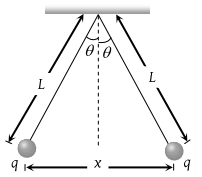(1) ${\left(\frac{{q}^{2}L}{2\pi {\epsilon }_{0}mg}\right)}^{\frac{1}{3}}$

(2) ${\left(\frac{q{L}^{2}}{2\pi {\epsilon }_{0}mg}\right)}^{\frac{1}{3}}$

(3) ${\left(\frac{{q}^{2}{L}^{2}}{4\pi {\epsilon }_{0}mg}\right)}^{\frac{1}{3}}$

(4) ${\left(\frac{{q}^{2}L}{4\pi {\epsilon }_{0}mg}\right)}^{\frac{1}{3}}$

Concept Questions :-

Coulomb's law
High Yielding Test Series + Question Bank - NEET 2020

Difficulty Level:

Three positive charges of equal value q are placed at the vertices of an equilateral triangle. The resulting lines of force should be sketched as in

(1)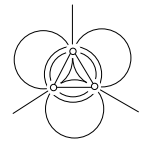(2)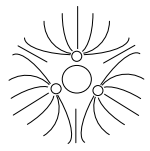(3)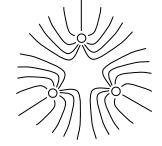(4)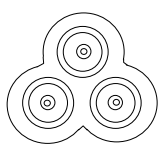Concept Questions :-

Electric field
High Yielding Test Series + Question Bank - NEET 2020

Difficulty Level:

Two equal charges are separated by a distance d. A third charge placed on a perpendicular bisector at x distance will experience maximum coulomb force when

(1) $x=\frac{d}{\sqrt{2}}$

(2) $x=\frac{d}{2}$

(3) $x=\frac{d}{2\sqrt{2}}$

(4) $x=\frac{d}{2\sqrt{3}}$

Concept Questions :-

Coulomb's law
High Yielding Test Series + Question Bank - NEET 2020

Difficulty Level:

An electric dipole is situated in an electric field of uniform intensity E whose dipole moment is p and moment of inertia is I. If the dipole is displaced slightly from the equilibrium position, then the angular frequency of its oscillations is

(1) ${\left(\frac{pE}{I}\right)}^{1/2}$

(2) ${\left(\frac{pE}{I}\right)}^{3/2}$

(3) ${\left(\frac{I}{pE}\right)}^{1/2}$

(4) ${\left(\frac{p}{IE}\right)}^{1/2}$

High Yielding Test Series + Question Bank - NEET 2020

Difficulty Level:

An infinite number of electric charges each equal to 5 nano-coulomb (magnitude) are placed along x-axis at x = 1 cm, x = 2 cm, x = 4 cm, x = 8 cm ………. and so on. In the setup if the consecutive charges have opposite sign, then the electric field in Newton/Coulomb at x = 0 is $\left(\frac{1}{4\pi {\epsilon }_{0}}=9×{10}^{9}N-{m}^{2}/{c}^{2}\right)$

(1) 12 × 104

(2) 24 × 104

(3) 36 × 104

(4) 48 × 104

Concept Questions :-

Electric field
High Yielding Test Series + Question Bank - NEET 2020

Difficulty Level:

A small sphere carrying a charge ‘q’ is hanging in between two parallel plates by a string of length L. Time period of pendulum is T0. When parallel plates are charged, the time period changes to T. The ratio T/T0 is equal to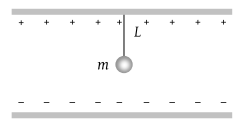(1) ${\left(\frac{g+\frac{qE}{m}}{g}\right)}^{1/2}$

(2) ${\left(\frac{g}{g+\frac{qE}{m}}\right)}^{3/2}$

(3) ${\left(\frac{g}{g+\frac{qE}{m}}\right)}^{1/2}$

(4) None of these

Concept Questions :-

Electric field
High Yielding Test Series + Question Bank - NEET 2020

Difficulty Level:

Three charges –q1, +q2 and –q3 are placed as shown in the figure. The x-component of the force on –q1 is proportional to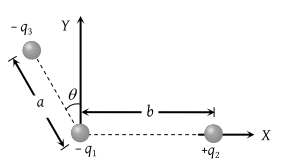(1) $\frac{{q}_{2}}{{b}^{2}}-\frac{{q}_{3}}{{a}^{2}}\mathrm{sin}\theta$

(2) $\frac{{q}_{2}}{{b}^{2}}-\frac{{q}_{3}}{{a}^{2}}\mathrm{cos}\theta$

(3) $\frac{{q}_{2}}{{b}^{2}}+\frac{{q}_{3}}{{a}^{2}}\mathrm{sin}\theta$

(4) $\frac{{q}_{2}}{{b}^{2}}+\frac{{q}_{3}}{{a}^{2}}\mathrm{cos}\theta$

Concept Questions :-

Electric field
High Yielding Test Series + Question Bank - NEET 2020

Difficulty Level:

Two-point charges +q and –q are held fixed at (–d, 0) and (d, 0) respectively of a (x, y) coordinate system. Then

(1) E at all points on the y-axis is along $\stackrel{^}{i}$

(2) The electric field $\stackrel{\to }{E}$ at all points on the x-axis has the same direction

(3) Dipole moment is 2qd directed along $\stackrel{^}{i}$

(4) Work has to be done in bringing a test charge from infinity to the origin

Concept Questions :-

Electric dipole
High Yielding Test Series + Question Bank - NEET 2020

Difficulty Level:

A point charge of 40 stat coulomb is placed 2 cm in front of an earthed metallic plane plate of large size. Then the force of attraction on the point charge is

(1) 100 dynes

(2) 160 dynes

(3) 1600 dynes

(4) 400 dynes

Concept Questions :-

Coulomb's law